### Home > PC > Chapter 4 > Lesson 4.2.1 > Problem4-73

4-73.
1. Simplify the following rational expressions into a single fraction. Homework Help ✎

1.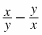2.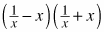3.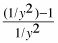4.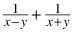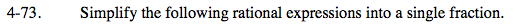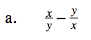$\text{Multiply the first fraction by }\frac{x}{x}\text{ and the second fraction}$

$\text{by }\frac{y}{y}\text{ to get a common denominator.}$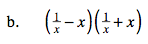Multiply out. Get a common denominator.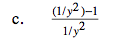The bottom fraction flips to the numerator:

$\left(\frac{1}{y^2}-1\right)\left(y^2\right)$

Distribute and simplify.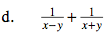$\frac{2x}{x^2-y^2}$